# Methods for Fit Mixed Effects Model

Select the method or formula of your choice.

## The mixed effects model and the log-likelihood

### The general form of the mixed effects model

Mixed effects models contain both fixed and random effects. The general form of the mixed effects model is:

y =+ Z1μ1 + Z2μ2 + ... + Zcμc + ε

### Notation

TermDescription
ythe n x 1 vector of response values
Xthe n x p design matrix for the fixed effect terms, pn
βa p x 1 vector of unknown parameters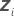the n x mi design matrix for therandom term in the model
μian mi x 1 vector of independent variables from N(0,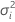)
εan n x 1 vector of independent variables from N(0,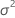)
nthe number of observations
pthe number of parameters in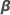cthe number of random terms in the model

### Variance-covariance matrix

Based on the model assumption for the general form of the mixed effects model, the response vector, y, has a multivariate normal distribution with mean vector and the following variance-covariance matrix:

V(σ2) = V(σ2, σ21, ... , σ2c) = σ2In + σ21Z1Z'1 + ... + σ2cZcZ'c

where

σ2 = (σ2, σ21, ... , σ2c)'

σ2, σ21, ... , σ2c are called variance components.

By factoring from the variance, you can find a representation of H(θ), which is in the computation of the log-likelihood of mixed effects models.

V(σ2) = σ2H(θ) = σ2[In + θ1Z1Z'1 + ... + θcZcZ'c]

### Notation

TermDescription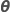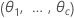θi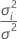, the ratio of the variance of therandom term over the error variance

### Log-likelihood

When the model contains a random factor, by default the unknown parameter estimates come from minimizing twice the negative of the restricted log-likelihood function. The minimization is equivalent to maximizing the restricted log-likelihood function. Minitab uses an iterative algorithm to minimize the restricted log-likelihood function. The function to minimize is:

### Notation

TermDescription
HIn + θ1Z1Z'1 + ... + θcZcZ'c
|H|the determinant of H
H-1the inverse of H
mithe number of levels for therandom termthe error variance component
Inthe identity matrix with n rows and columns

## Restricted Maximum Likelihood (REML) estimation

By default, Minitab calculates parameter estimates that maximize the restricted likelihood function, which is equivalent to minimizing the following function:
To minimize the function, Minitab differentiates the function with respect to β, σ2, and θi and sets the differentials equal to 0:

where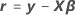Algebraic rearrangement of the first two equations to solve for the estimated parameters with respect to the differentiation give the following equations:
The derivative with respect to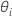cannot be explicitly solved for the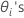. Minitab uses Newton's method to estimatewith the following steps:
1. Use the Minimum Norm Quadratic Unbiased Estimates (MINQUE)12 of the variance components to construct the initial values of σ2 and θi.
2. Estimate β and σ2 with the equations for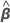and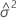.
3. Find θi with Newton's method to minimize L(β, σ2, θ).
4. Repeat steps 2 and 3 until convergence.
The converged solutions forare the variance ratio estimates. The variance component for therandom term is as follows:

### Notation

TermDescription
tr(·)the trace of the matrix
X'the transpose of X
1 Rao, C.R. (1971 a). Estimation of variance covariance components - MINQUE theory. Journal of Multivariate Analysis 1, 257–275.
2 Rao, C.R. (1971 b). Minimum variance quadratic unbiased estimation of variance components. Journal of Multivariate Analysis 1, 445–456.
By using this site you agree to the use of cookies for analytics and personalized content.  Read our policy